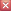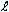Frequently Asked Questions To return to qm view, close tab or browserIf you have a question about the qm view, send it in an email to help@qmview.net and we will reply promptly.

 QUESTION:  What does rate of round-trip energy exchange mean? ANSWER:  The rate of something means how many times something occurs in a particular length of time (e.g. a heart rate might be 70 beats per minute). Energy exchange means transferring energy from one location to another. Energy can be exchanged between two locations by sound waves moving through air or a baseball moving between a pitcher and a catcher, but the energy we are concerned with is in the form of energy quanta such as photons that transfer energy over both subatomic and cosmic distances. A photon is described in the glossary. Energy can be exchanged between two locations (e.g. between Moscow and Paris or between two atoms in a solid body) by photons moving between the locations. Shortwave radio communication between two locations is an example of exchanging photon energy. The rate at which photons can make a round-trip transfer of energy between two locations depends on the distance between the locations and the speed of the photons traveling between the locations. In orthodox physics theory, the speed of photons moving between the locations is assumed to be always the same. A primary consequence of the quantum medium is that the speed of the photons moving between two locations on Earth changes as Earth's speed through the medium changes. The faster Earth moves through the medium the longer it takes for a round-trip radio signal between the two locations. If Earth's speed through the medium were to approach the speed of light through the medium, the time for a round-trip radio signal between the two locations would approach infinity and the rate of round-trip energy exchange between the two locations would approach zero. The speed of the signal might be very fast in one direction but it would approach zero in the other direction, so the rate of round-trip energy exchange would approach zero. QUESTION:  What does foreshorten mean? ANSWER:  In general it means that a body becomes shorter or appears to be shorter. In our discussions, the real, absolute foreshortening of a body will be in the direction of the body's absolute velocity through the quantum medium, and the observed, virtual foreshortening of a body will be in the direction of observed relative velocity. A spaceship moving lengthwise through the qm is foreshortened lengthwise. Its length is less than its length when at rest in the quantum medium. A spaceship moving sideways through the qm is not foreshortened lengthwise, but is foreshortened sideways. QUESTION:  What does different standards of time mean? ANSWER:  It means that the standards of time (i.e. the rates of clocks or other means of keeping time) are different for different observers or different reference frames. Clocks moving through the quantum medium run slower than clocks at rest in the qm. Therefore, if one reference frame is moving through the qm and another frame is at rest in the qm, the frames will have "different standards of time" unless the observers in the frames are aware of the clock slowing caused by motion through the qm and compensate for the slowing so they have the same standard of time. The logical standard of time is the "absolute second," which is 1 second according to a precise clock (e.g. atomic clock) at rest in the qm. The symbol for an absolute second is sa, as opposed to the symbol s, which means one second according to a clock that may be moving through the qm. If 100 clocks have different speeds through the qm, they will provide 100 different standards of time because the 1 s time duration of each clock will be different. Similarly, the standards of distance and mass also differ for reference frames with different speeds through the qm because the different speeds cause the standards of distance and mass (e.g. standard meter rods and standard kilogram blocks) to have different lengths and masses in each frame. On page 4 the distinction is made between observers(c) and observers(cr). The former are not aware that the rates of the clocks, the lengths of the standard measuring rods, and the masses of the standard masses in their reference frames depend on their speeds through the qm. But the latter are aware of these and other consequences of the qm and compensate for the consequences so that all the observers(cr) are using the same absolute standards of time, distance, and mass. After studying this website, it should be very clear to the reader why we need to distinguish between virtual units of time, distance, and mass (s, m, kg) and absolute units (sa, ma, kga), as we do on page 10 and Glossary. The sooner this becomes clear, the easier it will be for the reader to understand the quantum medium view. QUESTION:  What is the difference between speed and velocity? ANSWER:  The primary difference is that speed has only a magnitude, and velocity has both a magnitude and a direction. Even though the speed of a planet may be constant relative to the star it orbits, the velocity keeps changing because the direction of the planet's motion keeps changing. QUESTION:  How can the equations for the physical change ratio, rg, and the speed of light, cag, be correct if they predict rg=0 and cag=0 ca at the center of mass of a massive system like our galaxy or sun? ANSWER:  As with Newton's equation for determining the force, F, of gravity between two bodies having masses, m1 and m2, we cannot assume that the bodies' entire masses are located at their centers of mass in cases where the distance,, between the centers of mass is small relative to the sizes of the bodies. Newton's gravitational force equation:    F=m1m2G/2 rg and cag equations:    rg=/(+mG)     cag=rg2 For example, were we located a meter away from the center of mass of our sun (preferably in an air-conditioned room), Newton's equation (where the gravitational constant G equals 6.67E−11) would specify a gravitational force on our bodies of about 1E21 kg due to the sun's 2E30 kg mass. But the mass of the sun is not located at its center of mass. It is distributed around the center of mass so that the mass in one direction from the center is more or less the same as the mass in the opposite direction. Therefore the force on our bodies due to the mass in one direction will be about the same as the force in the opposite direction, and the net force on our bodies will be about zero.       Similarly, when using the rg equation in cases whereis small relative to the size of the masses, we cannot assume that a body's entire mass is at its center of mass. For our sun, which has a radius of about 2.3 light-second, we can estimate that the effective distance of the sun's mass from the sun's center might be about 1.2 light-second. Therefore, rg at the sun's center is about 0.99999588, and cag is about 0.99999177 ca (assuming that the sun's mass is the only influence on cag). Note that when using the rg equation, whereis in light-second units, G equals 2.47E−36 LS3/kga·sa2 rather than 6.67E−11 m3/kg·s2. QUESTION:  Does the quantum medium slow the speeds of planets, spaceships, or photons moving through it? Shouldn't the quantum medium create drag on bodies moving through it? ANSWER:  The quantum medium does not resist the motion of bodies moving through it because the bodies are comprised entirely of systems of oscillations in the qm. The qm view leads to the conclusion that the subatomic mass/energy (e.g. electrons, quarks, photons) comprising planets and other complex bodies are probably systems of oscillations in the qm. The energies of the subatomic quanta are due to the oscillations of the qm. Oscillations in the qm move freely through the qm, and the qm moves freely through oscillations in the qm.       A somewhat similar example is the motion of the energy in a sound wave through air or water. The wave moves freely through the medium, and the medium moves freely through the wave. However, unlike sound energy moving through air, water or other medium, the energy of oscillations moving through the qm is not appreciably dissipated in the medium. Photons that travel great distances for thousands of years appear not to lose any of their energy in the process. And the energy of the oscillations moving within electrons, nucleons, and other subatomic mass/energy is not appreciably dissipated in the medium. Thus, the qm should not be thought of as being like mediums comprised of mass/energy. QUESTION:  How much does the rate of an atomic clock vary during the year due to Earth's revolution around the sun? ANSWER:  Earth's absolute velocity, va, through the quantum medium is not constant due to Earth's revolution around the sun (and also the sun's motion around galaxy center, etc.). The cosmic microwave radiation dipole suggests that the sun's absolute velocity through the qm is about .0012 c toward the constellation Leo. Earth's velocity around the sun is .0001 c. Once a year this velocity relative to the sun is approximately in the direction of Leo, and six months later this velocity is away from Leo. Therefore, the change in Earth's absolute velocity during the year appears to be about .0002 c. Assuming this annual change in va for Earth, rv for Earth will change by approximately (1 − sqrt(1 − .0001^2)) or about 2E−8 per year. This will cause a clock rate change of about (2E−8 · 3E7 s/yr) or .6 s/yr. When the velocity of Earth around the sun is toward Leo, the rate of an atomic clock on Earth will be about .6 s/yr. slower than the rate six months later when Earth's va will be at a yearly minimum and the clock will have its fastest rate.

 To return to qm view, close tab or browser